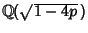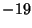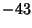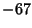## Lucky Number of Euler

A numbersuch that the Prime-Generating Polynomialis Prime for, 1, ...,. Such numbers are related to the Complex Quadratic Field in which the Ring of Integers is factorable. Specifically, the Lucky numbers of Euler (excluding the trivial case) are those numberssuch that the Quadratic Fieldhas Class Number 1 (Rabinowitz 1913, Le Lionnais 1983, Conway and Guy 1996).

As established by Stark (1967), there are only nine numberssuch that(the Heegner Numbers,,,,,,, and), and of these, only 7, 11, 19, 43, 67, and 163 are of the required form. Therefore, the only Lucky numbers of Euler are 2, 3, 5, 11, 17, and 41 (Le Lionnais 1983, Sloane's A014556), and there does not exist a better Prime-Generating Polynomial of Euler'sform.

References

Conway, J. H. and Guy, R. K. The Nine Magic Discriminants.'' In The Book of Numbers. New York: Springer-Verlag, pp. 224-226, 1996.

Le Lionnais, F. Les nombres remarquables. Paris: Hermann, pp. 88 and 144, 1983.

Rabinowitz, G. Eindeutigkeit der Zerlegung in Primzahlfaktoren in quadratischen Zahlkörpern.'' Proc. Fifth Internat. Congress Math. (Cambridge) 1, 418-421, 1913.

Sloane, N. J. A. Sequence A014556 in The On-Line Version of the Encyclopedia of Integer Sequences.'' http://www.research.att.com/~njas/sequences/eisonline.html.

Stark, H. M. A Complete Determination of the Complex Quadratic Fields of Class Number One.'' Michigan Math. J. 14, 1-27, 1967.